# Difference between revisions of "Distribution function"

of a random variable2010 Mathematics Subject Classification: Primary: 60E05 [MSN][ZBL]

The function of a real variabletaking at eachthe value equal to the probability of the inequality.

Every distribution function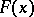has the following properties:

1)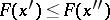when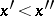;

2)is left-continuous at every;

3),. (Sometimes a distribution function is defined as the probability of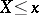; it is then right-continuous.)

In mathematical analysis, a distribution function is any function satisfying 1)–3). There is a one-to-one correspondence between the probability distributions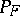on the-algebraof Borel subsets of the real line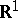and the distribution functions. This correspondence is as follows: For any interval,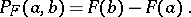Any functionsatisfying 1)–3) can be regarded as the distribution function of some random variable(e.g.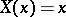) defined on the probability space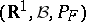.

Any distribution function can be uniquely written as a sum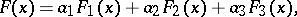where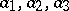are non-negative numbers with sum equal to 1, andare distribution functions such thatis absolutely continuous,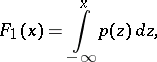is a "step-function" ,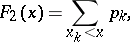where theare the points where"jumps" and the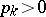are proportional to the size of these jumps, andis the "singular" component — a continuous function whose derivative is zero almost-everywhere.

Example. Let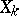,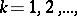be an infinite sequence of independent random variables assuming the values 1 and 0 with probabilities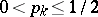and, respectively. Also, let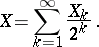Now:

1) if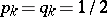for all, thenhas an absolutely-continuous distribution function (withfor, that is,is uniformly distributed on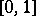);

2) if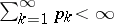, thenhas a "step" distribution function (it has jumps at all the dyadic-rational points in);

3) if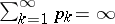andas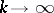, thenhas a "singular" distribution function.

This example serves to illustrate the theorem of P. Lévy asserting that the limit of an infinite convolution of discrete distribution functions can contain only one of the components mentioned above.

The "distance" between two distributionsandon the real line is often defined in terms of the corresponding distribution functionsand, by putting, for example,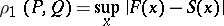orThe distribution functions of the probability distributions most often used (e.g. the normal, binomial and Poisson distributions) have been tabulated.

To test hypotheses concerning a distribution functionusing results of independent observations, one can use some measure of the deviation offrom the empirical distribution function (see Kolmogorov test; Kolmogorov–Smirnov test; Cramér–von Mises test).

The concept of a distribution function can be extended in a natural way to the multi-dimensional case, but multi-dimensional distribution functions are significantly less used in comparison to one-dimensional distribution functions.

For a more detailed treatment of distribution functions see Gram–Charlier series; Edgeworth series; Limit theorems.

How to Cite This Entry:
Distribution function. Encyclopedia of Mathematics. URL: http://encyclopediaofmath.org/index.php?title=Distribution_function&oldid=26407
This article was adapted from an original article by Yu.V. Prokhorov (originator), which appeared in Encyclopedia of Mathematics - ISBN 1402006098. See original article